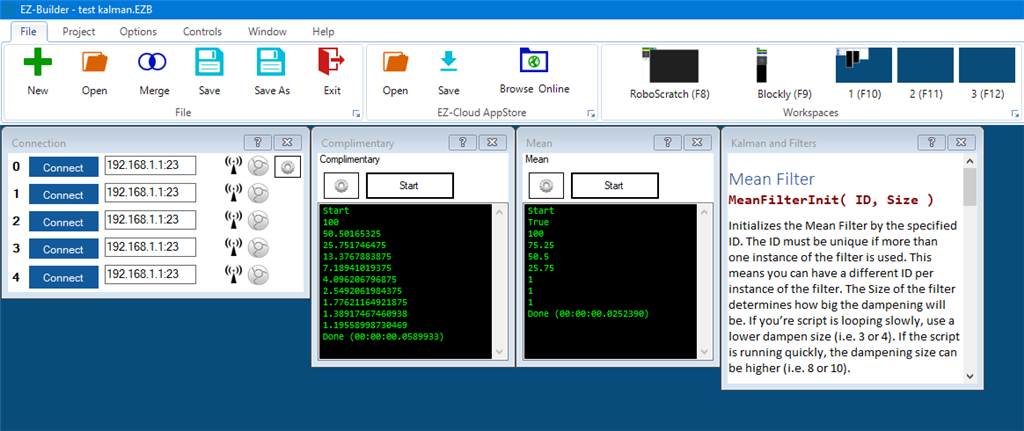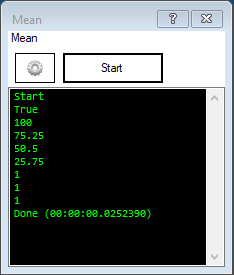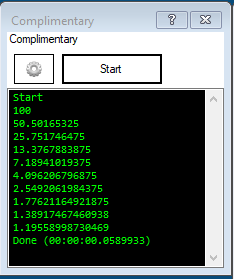Behavior Control
Created
Updated
Index
• Overview
• Artificial Intelligence
• Audio
• Camera
• Digital
• Games
• General
• Gps
• Graphs
• Hbridge
• I2c
• Infrared Distance
• Misc
• Mobile
• Pwm
• Rafiki
• Scripting
• Servo
• Third Party Robots
• Touch Tablet
• Ultrasonic Distance
• Virtual Reality

## Filters4. The installer will add this control to EZ-Builder.

This plugin will register new EZ-Script commands that can be used to dampen and filter values. For instance, if you are receiving data from a sensor to move servos, these filters will smooth the output to prevent the servo from being jerky.

There are currently two filters that can be used. In order to allow multiple instances of each filter, the commands have an ID. This means you can have multiple instances of the same filter and each instance can have an unique ID.Mean Filter Examples
Here is a short code that demonstrates the output of the mean filter if the values went from 100 to 1.

#### `Code:`

``# Initialize the filter with ID "test" and a dampen size of 4MeanFilterInit("test", 4)# Add the first value, which will be 100print(MeanFilterAdd("test", 100))# Add a bunch of 1's and print the output so you can see what the dampening looks likeprint(MeanFilterAdd("test", 1))print(MeanFilterAdd("test", 1))print(MeanFilterAdd("test", 1))print(MeanFilterAdd("test", 1))print(MeanFilterAdd("test", 1))print(MeanFilterAdd("test", 1))``

Output:Complimentary Filter
This code demonstrates the complimentary filter with an ID of "banana" and a dampen of 50%
`Code:# Init the filter with a dampening percentage of 50%ComplimentaryFilterInit("banana", 50)# The first value we will add is 100print( ComplimentaryFilterAdd("banana", 100))# Add and print a bunch of 1's so you can see how the dampening worksprint( ComplimentaryFilterAdd("banana", 1))print( ComplimentaryFilterAdd("banana", 1))print( ComplimentaryFilterAdd("banana", 1))print( ComplimentaryFilterAdd("banana", 1))print( ComplimentaryFilterAdd("banana", 1))print( ComplimentaryFilterAdd("banana", 1))print( ComplimentaryFilterAdd("banana", 1))print( ComplimentaryFilterAdd("banana", 1))print( ComplimentaryFilterAdd("banana", 1))`

Output: